# Mysterious Disappearance of an edge

Software version: Sage 10.0

The following codes can find all connected subgraphs.

g = graphs.CubeGraph(2)
subgraphs = list(g.connected_subgraph_iterator())
G=graphics_array([[v.plot() for v in subgraphs]],3,5)
G.show(axes=False, frame=False, figsize=5)


We will notice that there is an image missing an edge (due to the non-unique nature of iteration order, in my execution, it is the 6th one, and its image should be one edge instead of two isolated vertices).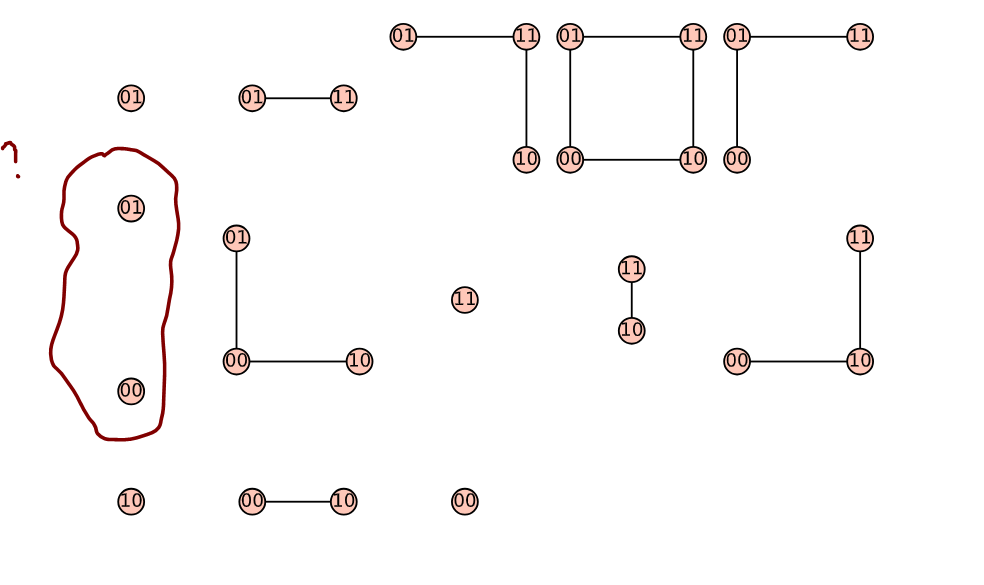The previous images might appear confusing because they are not separated by borders. I'm not know how to uniformly add nice borders to them (which is also the question I've been wanting to ask), otherwise, it would make the above issue more clear. The following images have been processed by using Inkscape. We can clearly see that the 6th image is missing an edge.

subgraphs.edges(sort=true,labels=False) # output: [('00', '01')]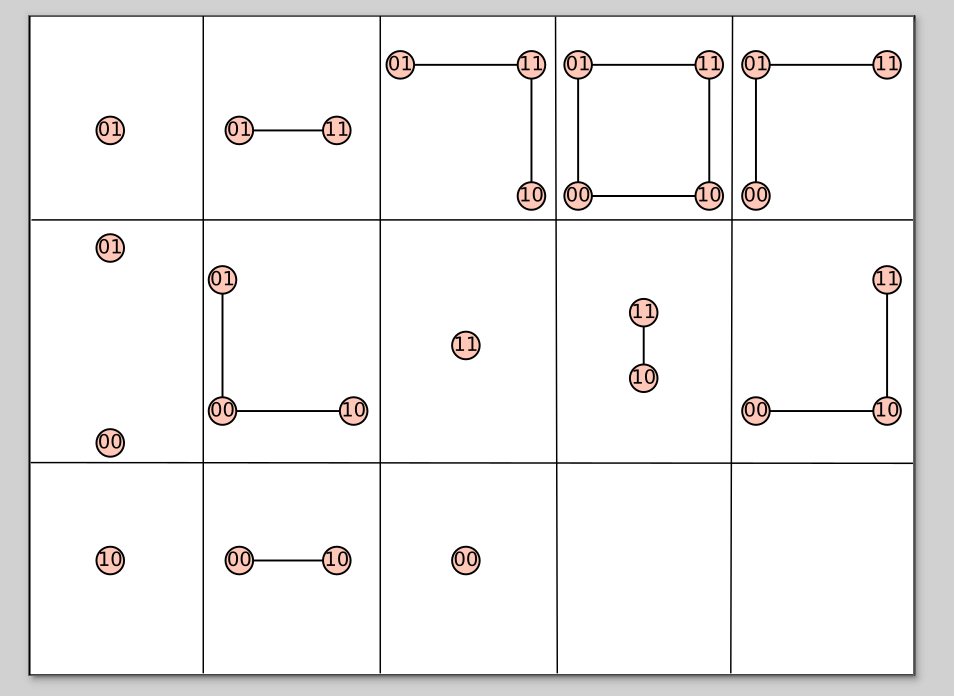edit retag close merge delete

Sort by » oldest newest most voted

One workaround is to add a frame by hand to each graph plot.

Here is one way to do that.

corners = [(-0.5, -0.5), (1.5, -0.5), (1.5, 1.5), (-0.5, 1.5)]
frame = polygon2d(corners, fill=False)
g = graphs.CubeGraph(2)
subgraphs = list(g.connected_subgraph_iterator())
G = graphics_array([[frame + v.plot() for v in subgraphs]], 3, 5)
G.show(axes=False, frame=False, figsize=(8, 5))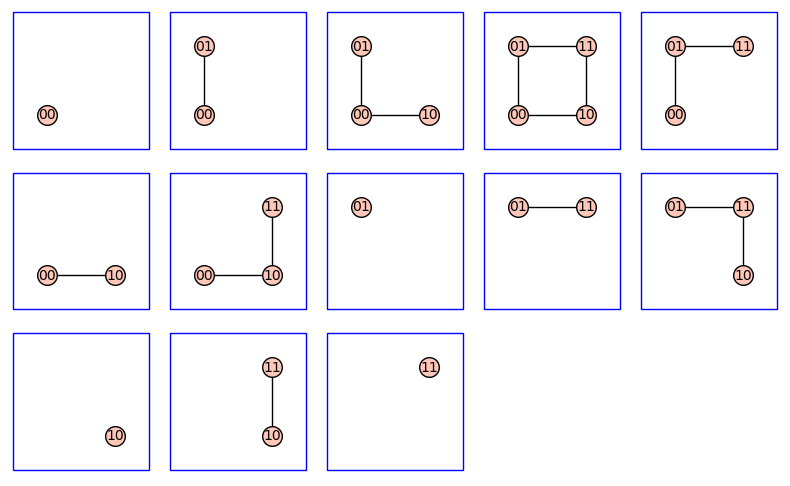more

1

I note that in this context, the (00, 10) edge is plotted. This hint that the problem may reside in the computation of the width of the graph...

NOTE : Not an answer,, but I needed to show a graph, which is not possible in a comment.

sage: G=graphics_array(list(map(lambda u:u.plot(graph_border=True),
graphs.CubeGraph(2).connected_subgraph_iterator())),
3, 5)
sage: G
Launched png viewer for Graphics Array of size 3 x 5


displays :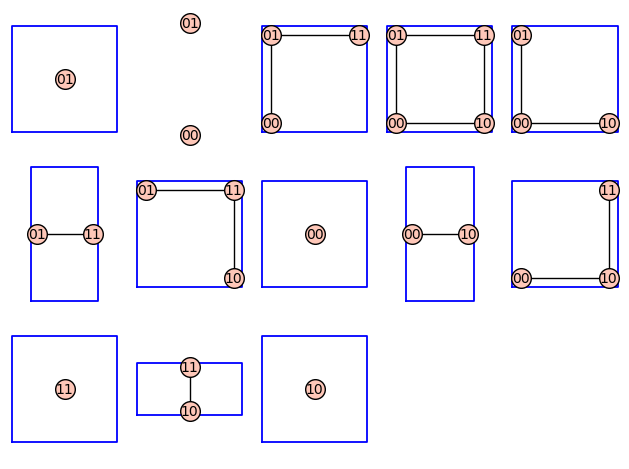One notes that the borders are "tight" ; this may be parameter-dependent (you'll have to look at the general plot parameters...).

One notes that the (00, 01) subgraph has neither visible edge nor border.

The following (intentional) blunder offers a hint :

sage: G=graphics_array(list(map(lambda u:u.plot(frame=True), graphs.CubeGraph(2).connected_subgraph_iterator())), 3, 5)
sage: G
Launched png viewer for Graphics Array of size 3 x 5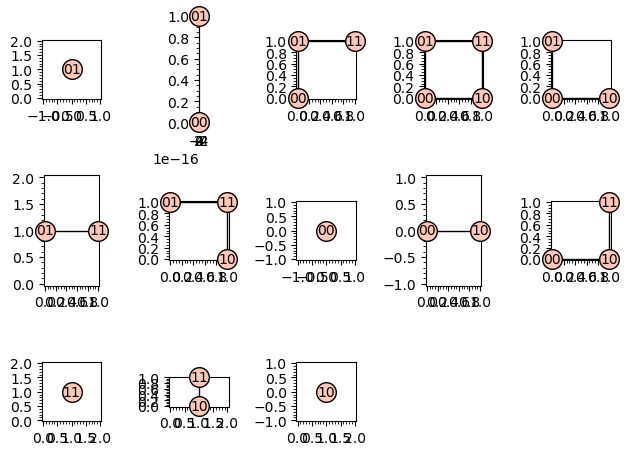(http://)

The suspicious supgraph has no width (whereas (01, 11) and (00, 10) have a non-zero height and (10, 11) has a width).

This smells of a bug in the .plot method (or possibly in the creation of the graph.CubeGraph layout). Would you mind explore it a bit and file an issue ?

EDIT :

sage: g=graphs.CubeGraph(2)
sage: gs=[u for u in g.connected_subgraph_iterator()]
sage: gs.get_pos()
{'11': (1.0, 1.0), '10': (1.0, 0.0)} # Plots okay
sage: gs.get_pos()
{'01': (6.123233995736766e-17, 1.0), '00': (0.0, 0.0)} # plots without width nor edge


the x coordinate of 01 is highly suspicious...

EDIT 2: I can confirm that the numerical value of the vertices positions is implied in the mechanism (pathogeny :-)) of this bug.

This is now issue #35905.

HTH,

more

I have asked this question before, although it is not directly related to this problem (may be the problem of tight borders). The reason behind it is not entirely clear to me. It seems to be an issue with plot, as you mentioned.